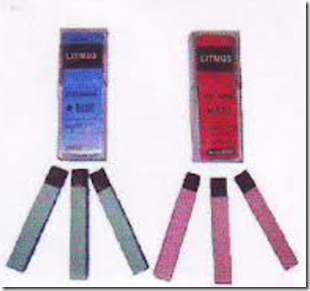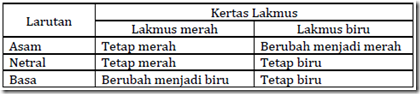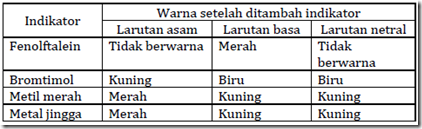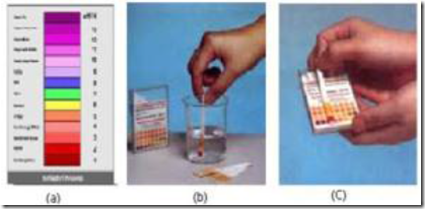Home > English > Water pH Measurement

Water pH Measurement

pH or acidity is used to express the degree of acidity (or alkalinity owned by a solution. That meant “acidity” is the concentration of hydrogen ions (H +) in water solvent. The pH values ​​ranging from 0 to 14. A solution is said to be neutral if it has pH = 7. The pH value> 7 indicates alkaline solution properties, while the pH value <7 indicates acidity.

The pH value of 7 is said to be neutral because the pure water of dissolved H + ions and OH- ions dissolved (as a sign of alkalinity) are at the same amount, which is 10-7 at equilibrium. The addition of H + ions dissolved compounds of an acid will push the equilibrium to the left (OH- ions are bound with H + to form water). As a result there is excess hydrogen ions and increase concentration.

Measurement of acid and alkaline compounds can be carried out using litmus paper, acid-base indicator (pH paper) and a pH meter.
a) Paper Litmus
There are two kinds of litmus paper which is used to identify acidic or alkaline compounds, namely litmus paper red and blue litmus paper.Figure 30. Paper Litmus

Table 19. Changes in the color of litmus paperFrom the table above it can be concluded that the acid solution will change the color of litmus paper blue to red, a neutral solution does not change the color of litmus paper, and an alkaline solution can turn litmus paper red to blue.

b) Acid Alkali Indicators
Acid-base indicator is a substance which gives a different color to the solution of acid and alkaline solution. With the difference in the color of the indicator can be used to determine whether a substance is acidic or alkaline. Consider the color of the indicator in acidic or alkaline solution below.

Table 20. Some indicators substances Acid AlkaliIndicators that can be used to recognize the nature of the acid or alkaline a solution and can be used to determine the price pH universal indicator. Here’s how to use universal indicator in determining the pH of a solutionFigure 31. Measurement of samples with pH paper (a) universal indicator image, (b) universal indicator used by dipping a universal indicator to limit the colors to the solution pH to be determined, will look at the color change indicator paper, (c) and then match changes color with the color indicator on the box. And the pH of the solution can be determined."Tasikmalaya, Indonesia"